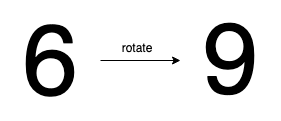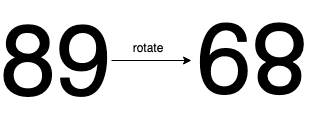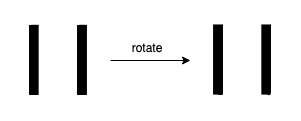##### Welcome to Subscribe On Youtube

Formatted question description: https://leetcode.ca/all/1056.html

# 1056. Confusing Number

Easy

## Description

Given a number N, return true if and only if it is a confusing number, which satisfies the following condition:

We can rotate digits by 180 degrees to form new digits. When 0, 1, 6, 8, 9 are rotated 180 degrees, they become 0, 1, 9, 8, 6 respectively. When 2, 3, 4, 5 and 7 are rotated 180 degrees, they become invalid. A confusing number is a number that when rotated 180 degrees becomes a different number with each digit valid.

Example 1:Input: 6

Output: true

Explanation:

We get 9 after rotating 6, 9 is a valid number and 9!=6.

Example 2:Input: 89

Output: true

Explanation:

We get 68 after rotating 89, 86 is a valid number and 86!=89.

Example 3:Input: 11

Output: false

Explanation:

We get 11 after rotating 11, 11 is a valid number but the value remains the same, thus 11 is not a confusing number.

Example 4:Input: 25

Output: false

Explanation:

We get an invalid number after rotating 25.

Note:

1. 0 <= N <= 10^9
2. After the rotation we can ignore leading zeros, for example if after rotation we have 0008 then this number is considered as just 8.

## Solution

To get the rotated number of the given number, for each digit from the right to the left, rotate the digit and append the digit to the rotated number. If a digit is invalid for rotation, return false. If the rotated number is valid, then check whether the rotated number equals the given number, and return true if and only if the rotated number doesn’t equal the given number.

• class Solution {
public boolean confusingNumber(int N) {
String num = String.valueOf(N);
int length = num.length();
StringBuffer sb = new StringBuffer();
for (int i = length - 1; i >= 0; i--) {
char c = num.charAt(i);
if (c != '0' && c != '1' && c != '8' && c != '6' && c != '9')
return false;
sb.append(rotate(c));
}
String rotate = sb.toString();
return !num.equals(rotate);
}

public char rotate(char c) {
if (c == '6' || c == '9')
return (char) ('6' + '9' - c);
else
return c;
}
}

• // OJ: https://leetcode.com/problems/confusing-number/
// Time: O(lgN)
// Space: O(1)
class Solution {
public:
bool confusingNumber(int n) {
int m = {0,1,-1,-1,-1,-1,9,-1,8,6}, r = 0, num = n;
while (n) {
if (m[n % 10] == -1) return false;
r = r * 10 + m[n % 10];
n /= 10;
}
return num != r;
}
};

• class Solution:
def confusingNumber(self, n: int) -> bool:
x, y = n, 0
d = [0, 1, -1, -1, -1, -1, 9, -1, 8, 6]
while x:
x, v = divmod(x, 10)
if d[v] < 0:
return False
y = y * 10 + d[v]
return y != n


• func confusingNumber(n int) bool {
d := []int{0, 1, -1, -1, -1, -1, 9, -1, 8, 6}
x, y := n, 0
for x > 0 {
v := x % 10
if d[v] < 0 {
return false
}
y = y*10 + d[v]
x /= 10
}
return y != n
}